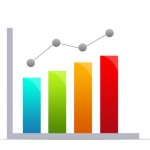# Excel draw stacked chart with empty future dataSolution to draw Excel stacked chart with empty future data First of all, it is true that Excel stacked chart would consider empty data as "0" instead of ignoring them like the case in non-stacked chart, it is the behavior that cannot be changed. In order not to change data range every week, it is better to set dynamic data range so that if there is no data in December 19, the data range (both data and x-axis label) is resized to December 12. You can find the solution in the below art...
More

# Excel graph dynamic data rangeThis Excel tutorial explains how to set Excel graph dynamic data range. You may also want to read: Excel dynamic Data Validation list Excel Dynamic Print Area Excel dynamic data range Excel graph dynamic data range The purpose of Excel graph dynamic data range is to select data range based on how many data you have input instead of using a fixed range. Assume that you have the data set below. You can draw a graph from January to October easily by selecting A1 to B11, bu...
More